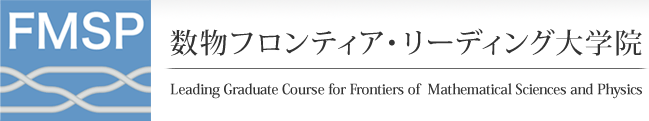#English

ホーム > 研究集会、講演会等 > 詳細内容

# 研究集会、講演会等

## Monte Carlo Methods for Partial Differential Equations: Computing Permeability

Michael Mascagni (Florida State University)
2013年2月21日 15:00-16:00+epsilon

Abstract: We present a brief overview of Monte Carlo methods for the solution of elliptic and parabolic partial differential equations (PDEs).  We begin with a review of the Feynman-Kac formula, and its use in the probabilistic representation of the solutions of elliptic and parabolic PDEs. We then consider some specific Monte Carlo methods used for obtaining the solution of simple elliptic partial differential equations (PDEs) as part of exterior boundary value problems that arise in electrostatics and flow through porous media. These Monte Carlo methods use Feynman-Kac to represent the solution of the elliptic PDE at a point as the expected value of functionals of Brownian motion trajectories started at the point of interest. We discuss the rapid solution of these equations, in complex exterior geometries, using both the "walk on spheres" and "Greens function first-passage" algorithms. We then concentrate on methods for quickly computing the isotropic permeability using the "unit capacitance" and "penetration depth'' methods. The first of these methods, requires computing a linear functional of the solution to an exterior elliptic PDE. Both these methods for computing permeability are simple, and provide accurate solutions in a few seconds on laptop-scale computers.  We then conclude with a brief look at other Monte Carlo methods and problems that arise on related application areas.